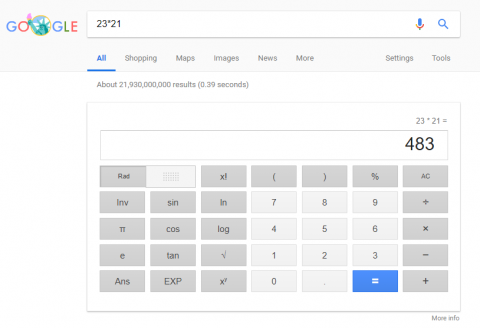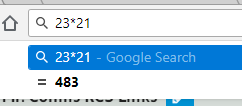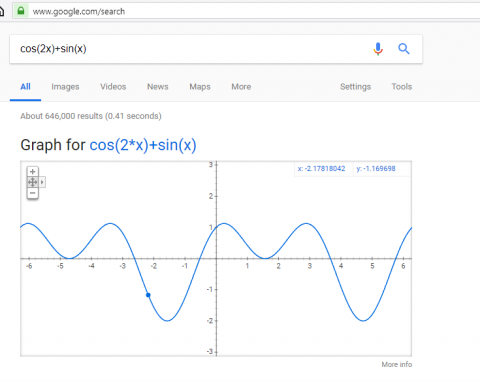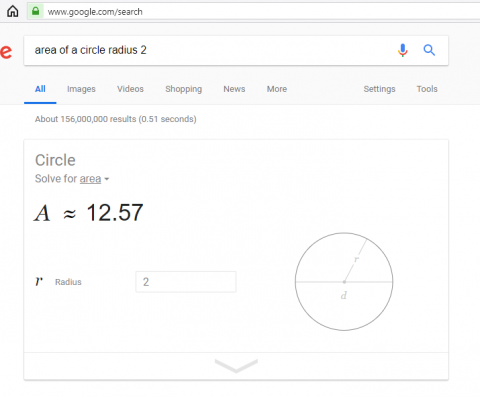How to use the calculator built in to Google.This may be an old trick, but it’s still very useful. When performing a Google search, you can instead type a problem to solve, such as 23*21 like in the example pic above. Google will then switch to calculator mode and show you the answer. You can continue with more problems from there if you need. Another way to launch the calculator is to complete a Google search for the word calculator.

If you perform the search from the URL in Google Chrome, the answer will automatically show without actually performing the search.This works the same way on Chromebooks when you use the search key.

Google search is also able to graph equations:And can graph multiple equations on the same chart if you separate the equations with a comma.

Finally, the Google calculator can complete some geometric calculations. For example, searching for area of a circle with a radius of 2 will calculate the area.